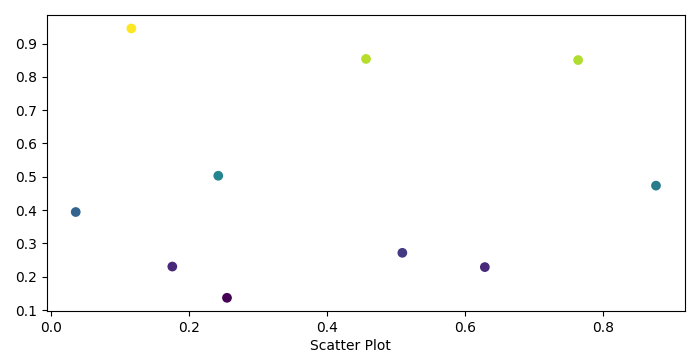# Adding caption below X-axis for a scatter plot using Matplotlib

To add caption below X-axis for a scatter plot, we can use text() method for the current figure.

## Steps

• Create x and y data points using numpy.

• Create a new figure or activate an existing figure using figure() method.

• Plot the scatter points with x and y data points.

• To add caption to the figure, use text() method.

• To display the figure, use show() method.

## Example

import numpy as np
from matplotlib import pyplot as plt
plt.rcParams["figure.figsize"] = [7.00, 3.50]
plt.rcParams["figure.autolayout"] = True
x = np.random.rand(10)
y = np.random.rand(10)
fig = plt.figure()
plt.scatter(x, y, c=y)
fig.text(.5, .0001, "Scatter Plot", ha='center')
plt.tight_layout()
plt.show()

## Output